Courses

# Thermodynamics MCQ - 4 (Advanced)

## 10 Questions MCQ Test Chemistry for JEE Advanced | Thermodynamics MCQ - 4 (Advanced)

Description
This mock test of Thermodynamics MCQ - 4 (Advanced) for JEE helps you for every JEE entrance exam. This contains 10 Multiple Choice Questions for JEE Thermodynamics MCQ - 4 (Advanced) (mcq) to study with solutions a complete question bank. The solved questions answers in this Thermodynamics MCQ - 4 (Advanced) quiz give you a good mix of easy questions and tough questions. JEE students definitely take this Thermodynamics MCQ - 4 (Advanced) exercise for a better result in the exam. You can find other Thermodynamics MCQ - 4 (Advanced) extra questions, long questions & short questions for JEE on EduRev as well by searching above.
QUESTION: 1

Solution:
QUESTION: 2

Solution:
QUESTION: 3

### For a reversible reaction at constant temperature and at constant pressure the equilibrium composition of reaction mixture corresponds to the lowest point on Gibbis energy Vs progress of reaction diagrams as shown. At equilibrium Gibbs energy of reaction is equal to Zero.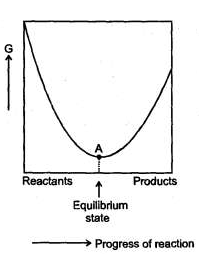For a reaction M2 O(s)→ 2M(S) →2M(s) + 1/2O2(g) ΔH = 30 KJ/mol and ΔS = 0.07 KJ/mol /K at latm. The reaction would not be spontaneous at  temperatures

Solution:
QUESTION: 4

The contributions of both heat (enthalpy) and randomness (entropy) shall be considered to the overall spontaneity of a process. When deciding about the spontaneity of a chemical reaction or other process, we define a quantity called the Gibb's energy change (ΔG).

ΔG = ΔH – TΔS

where,        ΔH = Enthalpy change ; ΔS = Entropy change;

T = Temperature in kelvin.

If ΔG < 0, Process is spontaneous ; ΔG = 0, Process is at equilibrium, AG > 0, Process is non-spontaneous.

Q.

For the change

H20 (s, 273 K, 2 atm) → H20 (l, 273K, 2 atom),

choose the correct option.

Solution:

H2O (s) →H2O ( l ),

As P ­ , more H2O ( l ) is formed.
At P = 1 atm, Δ G = 0.

QUESTION: 5

The contributions of both heat (enthalpy) and randomness (entropy) shall be considered to the overall spontaneity of a process. When deciding about the spontaneity of a chemical reaction or other process, we define a quantity called the Gibb's energy change (ΔG).

ΔG = ΔH – TΔS

where,        ΔH = Enthalpy change ; ΔS = Entropy change;

T = Temperature in kelvin.

If ΔG < 0, Process is spontaneous ; ΔG = 0, Process is at equilibrium, AG > 0, Process is non-spontaneous.

5 mol of liquid water is compressed from 1 bar to 10 bar at constant temperature. Change in Gibb's energy (ΔG) in Joule is t [Density of water = 1000 kg/m3].

Solution:

Δ G = V (P2 - P1) = 5 × 18 × 10-6 (101 - 1) × 105 Joule = 5 × 18 × 10-1 × 102 = 900 Joule.

QUESTION: 6

The contributions of both heat (enthalpy) and randomness (entropy) shall be considered to the overall spontaneity of a process. When deciding about the spontaneity of a chemical reaction or other process, we define a quantity called the Gibb's energy change (ΔG).

ΔG = ΔH – TΔS

where,        ΔH = Enthalpy change ; ΔS = Entropy change;

T = Temperature in kelvin.

If ΔG < 0, Process is spontaneous ; ΔG = 0, Process is at equilibrium, AG > 0, Process is non-spontaneous.

Q.

Quick lime, Ca0 is produced by heating limestone, CaC03 to drive off C02 gas.

CaC03 (s) → CaO (s) + C02 (g), (ΔH°) = 180 KJ, ΔS° = 150 J/K.

Assuming that variation of enthalpy change and entropy change with temperature to be negligible, choose the correct option :

Solution: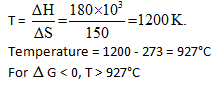QUESTION: 7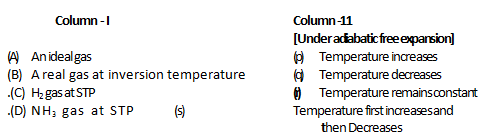Solution:
QUESTION: 8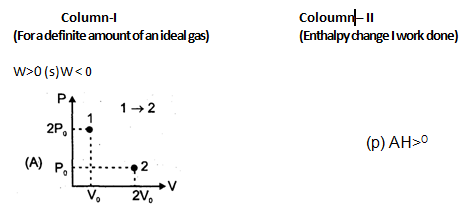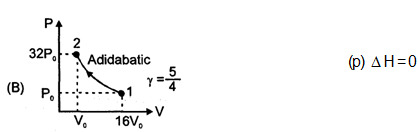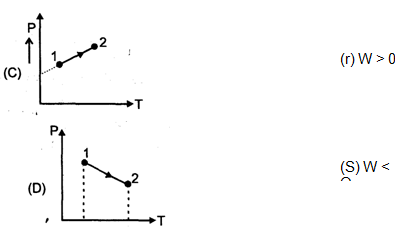Solution:
QUESTION: 9

The feasibility of a chemical reaction can be explained based on H, S and G, so answer the following :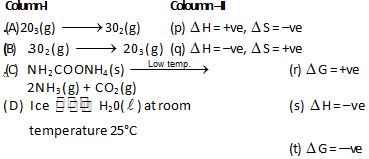Solution:
QUESTION: 10

Match the following :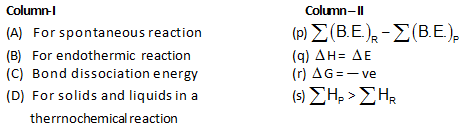Solution:

All the matching is obvious from the fact see the formula.